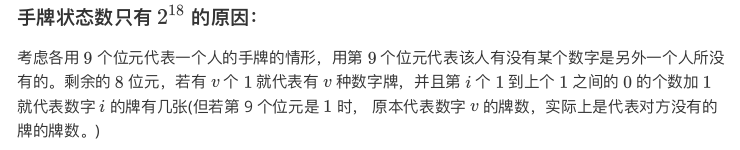# cometoj_1C 复读游戏

Contents

### 题目：

AA 发明了一个和复读有关的双人游戏，游戏的内容如下：

两人手上各有 张牌，每张牌上面个写了一个整数数字，两人都知道对方的每张牌上写的数字是什么。
游戏开始后，两人轮流出一张手上的牌，出出去的牌不会再收回。若某个人出的牌和上一家最后一次出的牌牌上写的数字一样，就输了。(注：先手在游戏最开始出的第一张牌无论是什么都不算输。)
若两人都把牌出完了但没有发生上述情况，则是平手。

AA 找了 dreamoon 一起玩这个游戏，并且由 AA 当先手。现在告诉你 AA 和 dreamoon 手上的牌上写的数字各是什么，请问谁有必胜策略呢？

### 思路：

手玩了半天也没发现什么结论（不过出题人好像有个只能暴力验证的结论），注意到 ，所以可以尝试比较暴力的 DP。
虽然没有找到结论，但是还是有一些性质可以利用的：
1. 编号不影响答案。如 相同。
2. 如果 AA 有某种 dreamoon 没有的牌，牌的编号可以任意，甚至不同的牌的编号都能变成同一种（只有这种编号 dreamoon 也没有），两人调换也成立。如 在决策上是相同的。
所以通过离散，牌的编号就能压缩成 ，这样手牌的状态数就不大了。按照出题人的算法状态数只有 ，但我觉得应该比这个值还要小。出题人的算法如下：比如 应该是等价的，因为编号不影响结果。
每次再记录上一次打的牌，总的状态就是 ，总的复杂度应该是 ，具体实现可以用 map 去映射一个 vector（每次映射前可以先排一次序，这样状态会更少），反正也跑的挺快的。

### 代码：

#include <bits/stdc++.h>
#define rep(i, a, b) for(int i(a), i##_END_(b); i <= i##_END_; i++)
#define drep(i, a, b) for(int i(a), i##_END_(b); i >= i##_END_; i--)
using namespace std;
template<class T> inline bool tomax(T &a, T b) {return a < b ? a = b, 1 : 0;}
template<class T> inline bool tomin(T &a, T b) {return b < a ? a = b, 1 : 0;}
typedef long long ll;
typedef double db;
typedef pair<int, int> pii;
#define fi first
#define se second
#define mr make_pair

template<class T>
inline void rd(T &a) {
#define gc getchar()
char c;
bool f = false;
for(c = gc; !isdigit(c); c = gc) f |= (c == '-');
for(a = 0; isdigit(c); c = gc) a = (a << 1) + (a << 3) + c - '0';
if(f) a = -a;
#undef gc
}

map<vector<pii>, short> mp;
int dfs(vector<pii> v, pii las) {
int id = 0;
sort(v.begin(), v.end());
rep(i, 0, 9) if(v[i] == las) id = i;
if(mp[id][v]) return mp[id][v];
int cnt = 0;
rep(i, 0, 9) cnt += v[i].fi + v[i].se;
if(cnt == 0) return mp[id][v] = 3;
int ans = 2;
rep(i, 0, 9) {
if(i == id) continue;
if(v[i].fi == 0) continue;
vector<pii> t;
rep(j, 0, 9) t.push_back(mr(v[j].se, v[j].fi - (i == j)));
int k = dfs(t, mr(v[i].se, v[i].fi - 1));
if(k == 2) {ans = 1; break;}
if(k == 3) ans = 3;
}
return mp[id][v] = ans;
}

int cnt;
int main() {
// freopen("data.in", "r", stdin);
int cas, n, a;
rd(cas);
while(cas--) {
rd(n);
memset(cnt, 0, sizeof cnt);
rep(d, 0, 1)
rep(i, 1, n) {
rd(a);
cnt[a][d]++;
}
vector<pii> v;
int x = 0, y = 0;
rep(i, 1, 20) {
if(cnt[i] && cnt[i])
v.push_back(mr(cnt[i], cnt[i]));
else
x += cnt[i], y += cnt[i];
}
while(v.size() < 8) v.push_back(mr(0, 0));
v.push_back(mr(0, y));
v.push_back(mr(x, 0));
int k = dfs(v, mr(0, 0));
if(k == 1) puts("AA wins");
if(k == 2) puts("dreamoon wins");
if(k == 3) puts("Draw");
}
return 0;
}